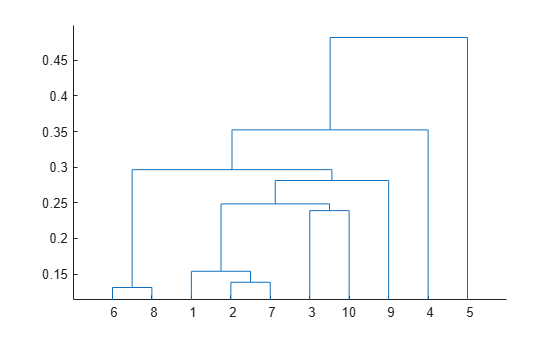# inconsistent

Inconsistency coefficient

## Syntax

``Y = inconsistent(Z)``
``Y = inconsistent(Z,d)``

## Description

example

````Y = inconsistent(Z)` returns the inconsistency coefficient for each link of the hierarchical cluster tree `Z` generated by the `linkage` function. `inconsistent` calculates the inconsistency coefficient for each link by comparing its height with the average height of other links at the same level of the hierarchy. The larger the coefficient, the greater the difference between the objects connected by the link. For more information, see Algorithms.```

example

````Y = inconsistent(Z,d)` returns the inconsistency coefficient for each link in the tree `Z` by searching to a depth `d` below each link.```

## Examples

collapse all

Examine an inconsistency coefficient calculation for a hierarchical cluster tree.

Load the `examgrades` data set.

`load examgrades`

Create a hierarchical cluster tree.

`Z = linkage(grades);`

Create a matrix of inconsistency coefficient information using `inconsistent`. Examine the information for the 84th link.

```Y = inconsistent(Z); Y(84,:)```
```ans = 1×4 7.2741 0.3624 3.0000 0.5774 ```

The fourth column of `Y` contains the inconsistency coefficient, which is computed using the mean in the first column of `Y` and the standard deviation in the second column of `Y`.

Because the rows of `Y` correspond to the rows of `Z`, examine the 84th link in `Z`.

`Z(84,:)`
```ans = 1×3 190.0000 203.0000 7.4833 ```

The 84th link connects the 190th and 203rd clusters in the tree and has a height of `7.4833`. The 190th cluster corresponds to the link of index $190-120=70$, where 120 is the number of observations. The 203rd cluster corresponds to the 83rd link.

By default, `inconsistent` uses two levels of the tree to compute `Y`. Therefore, it uses only the 70th, 83rd, and 84th links to compute the inconsistency coefficient for the 84th link. Compare the values in `Y(84,:)` with the corresponding computations by using the link heights in `Z`.

`mean84 = mean([Z(70,3) Z(83,3) Z(84,3)])`
```mean84 = 7.2741 ```
`std84 = std([Z(70,3) Z(83,3) Z(84,3)])`
```std84 = 0.3624 ```
`inconsistent84 = (Z(84,3)-mean84)/std84`
```inconsistent84 = 0.5774 ```

Create the sample data.

```X = gallery('uniformdata',[10 2],12); Y = pdist(X);```

Generate the hierarchical cluster tree.

`Z = linkage(Y,'single');`

Generate a dendrogram plot of the hierarchical cluster tree.

`dendrogram(Z)`Compute the inconsistency coefficient for each link in the cluster tree Z to depth 3.

`W = inconsistent(Z,3)`
```W = 9×4 0.1313 0 1.0000 0 0.1386 0 1.0000 0 0.1463 0.0109 2.0000 0.7071 0.2391 0 1.0000 0 0.1951 0.0568 4.0000 0.9425 0.2308 0.0543 4.0000 0.9320 0.2395 0.0748 4.0000 0.7636 0.2654 0.0945 4.0000 0.9203 0.3769 0.0950 3.0000 1.1040 ```

## Input Arguments

collapse all

Agglomerative hierarchical cluster tree, specified as a numeric matrix returned by `linkage`. `Z` is an (m – 1)-by-3 matrix, where m is the number of observations. Columns 1 and 2 of `Z` contain cluster indices linked in pairs to form a binary tree. `Z(I,3)` contains the linkage distances between the two clusters merged in row `Z(I,:)`.

Data Types: `single` | `double`

Depth, specified as a positive integer scalar. For each link k, `inconsistent` calculates the corresponding inconsistency coefficient using all the links in the tree within `d` levels below k.

Data Types: `single` | `double`

## Output Arguments

collapse all

Inconsistency coefficient information, returned as an (m – 1)-by-4 matrix, where the (m – 1) rows correspond to the rows of `Z`. This table describes the columns of `Y`.

ColumnDescription

1

Mean of the heights of all the links included in the calculation

2

Standard deviation of the heights of all the links included in the calculation

3

Number of links included in the calculation

4

Inconsistency coefficient

Data Types: `double`

## Algorithms

For each link k, the inconsistency coefficient is calculated as

`$Y\left(k,4\right)=\left(Z\left(k,3\right)-Y\left(k,1\right)\right)/Y\left(k,2\right),$`

where `Y` is the inconsistency coefficient information for links in the hierarchical cluster tree `Z`.

For links that have no further links below them, the inconsistency coefficient is set to 0.

 Jain, A., and R. Dubes. Algorithms for Clustering Data. Upper Saddle River, NJ: Prentice-Hall, 1988.

 Zahn, C. T. “Graph-theoretical methods for detecting and describing Gestalt clusters.” IEEE Transactions on Computers. Vol. C-20, Issue 1, 1971, pp. 68–86.